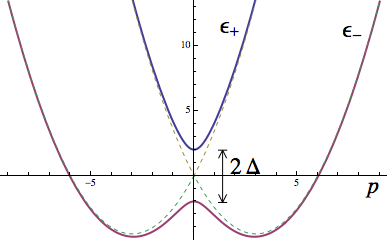# Spin-orbit coupled Hamiltonian

April 28, 2013

For applications in many parts of condensed matter physics and cold atoms physics, we use what is known as the Rashba spin-orbit coupled Hamiltonian. This Hamiltoninan is so-named because it couples momentum $\mathbf{p}$ to the spin $\mathbf{S}=\frac12\sigma$ where $\sigma = (\sigma_x,\sigma_y,\sigma_z)$ are the Pauli matrices and $\mathbf{p}=(p_x,p_y,p_z)$ is a vector of momentum operators:

$m$ is the mass, $\alpha$ is the spin-orbit coupling strength, and $\Delta$ is some Zeeman field (it acts as magnetic field on the spin).

In this post, we go through the calculation of the energy spectrum and eigenvectors – a straight forward exercise in undergraduate linear algebra.

First of all, instead of the normal method of finding eigenvectors, we note that we can rewrite this Hamiltonian in the form

where $\mathbf{b}(p) = (\alpha p_y, -\alpha p_x, \Delta)$. Now, $\mathbf{b}(p)$ represents a point on the Bloch sphere, and so we expect the eigenvectors to be parallel and anti-parallel to this vector. The energies in this case are very straight forward and amount to the positive and negative of $\lvert\mathbf{b}(p)\rvert$:

With these eigenvalues, it is a straight forward exercise in linear algebra to find the eigenvectors. After a bit of algebra, the eigenvectors of $H$ in terms of the eigenvectors of $\sigma_z$ ( $\sigma_z\left\lvert\uparrow\right\rangle = \left\lvert\uparrow\right\rangle$ and $\sigma_z\left\lvert\uparrow\right\rangle = -\left\lvert\uparrow\right\rangle$ ) are

where we have defined $\phi$ by $p_y+ip_x = p e^{i\phi}$. Note that when $p_{x,y} \rightarrow -p_{x,y}$, the occupations stay the same. However, if we just look at one energy, $\epsilon_-(p)$ the ground state energy, we see that the state we get when $p_{x,y} \rightarrow -p_{x,y}$ is almost orthogonal to the original state.The energy bands themselves look like the figure on the right where the vertical axis is energy (and for this particular example, $m=1$, $\alpha = 3$, and $\Delta=2$). Interestingly, the introduction of $\Delta$ actually causes the gap to open up – the dotted lines are for when $\Delta=0$.

Now, if we have a bunch of fermions filling up these energies, if we set the chemical potential to be in the gap, we would find that the only excitations would states that are spin-locked to the momentum.

Many things can be done with this Hamiltonian to interesting effect. It finds its way into cold atom physics as well as condensed matter.

Spin-orbit coupled Hamiltonian - April 28, 2013 - Justin H. Wilson